# NCERT Solutions For Class 10 Maths Chapter 3 Exercise 3.2

## Chapter 3 Ex.3.2 Question 1

Form the pair of linear equations in the following problems and find their Solutions graphically.

(i) $$10$$ students of Class X took part in a Mathematics quiz. If the number of girls is $$4$$ more than the number of boys, find the number of boys and girls who took part in the quiz.

(ii) $$5$$ pencils and $$7$$ pens together cost ₹ $$50,$$ whereas $$7$$ pencils and $$5$$ pens together cost ₹ $$46.$$ Find the cost of one pencil and that of one pen.

### Solution

(i)

What is Known?

(i) Number of students took part in Quiz $$= 10$$

(ii) Number of girls is $$4$$ more than number of boys

What is Unknown?

Finding Solutions graphically for the given situation.

Reasoning:

Assuming the number of boys as $$x$$ and the number of girls as $$y,$$ two linear equations can be formed for the above situation.

Steps:

Total number of boys and girls is:

$x + y = 10$

Number of girls is $$4$$ more than the number of boys,

Mathematically:

\begin{align}y &= x + 4\\ - x + y &= 4\end{align}

Algebraic representation where $$x$$ and $$y$$ are the number of boys and girls respectively.

\begin{align}x + y &= 10 \,\dots(1)\\- x + y &= 4\,\dots(2) \end{align}

Therefore, the algebraic representation for equation 1 is:

\begin{align}x + y &= 10\\y &= 10-x\end{align}

And, the algebraic representation is for equation 2 is:

\begin{align} - x + y& = 4\\y &= x + 4\end{align}

Let us represent these equations graphically. For this, we need at least two solutions for each equation. We give these solutions in table shown below.

 $$x$$ $$2$$ $$7$$ $$y = 10 - x$$ $$8$$ $$3$$

 $$x$$ $$1$$ $$4$$ $$y = x + 4$$ $$5$$ $$8$$

The graphical representation is as follows.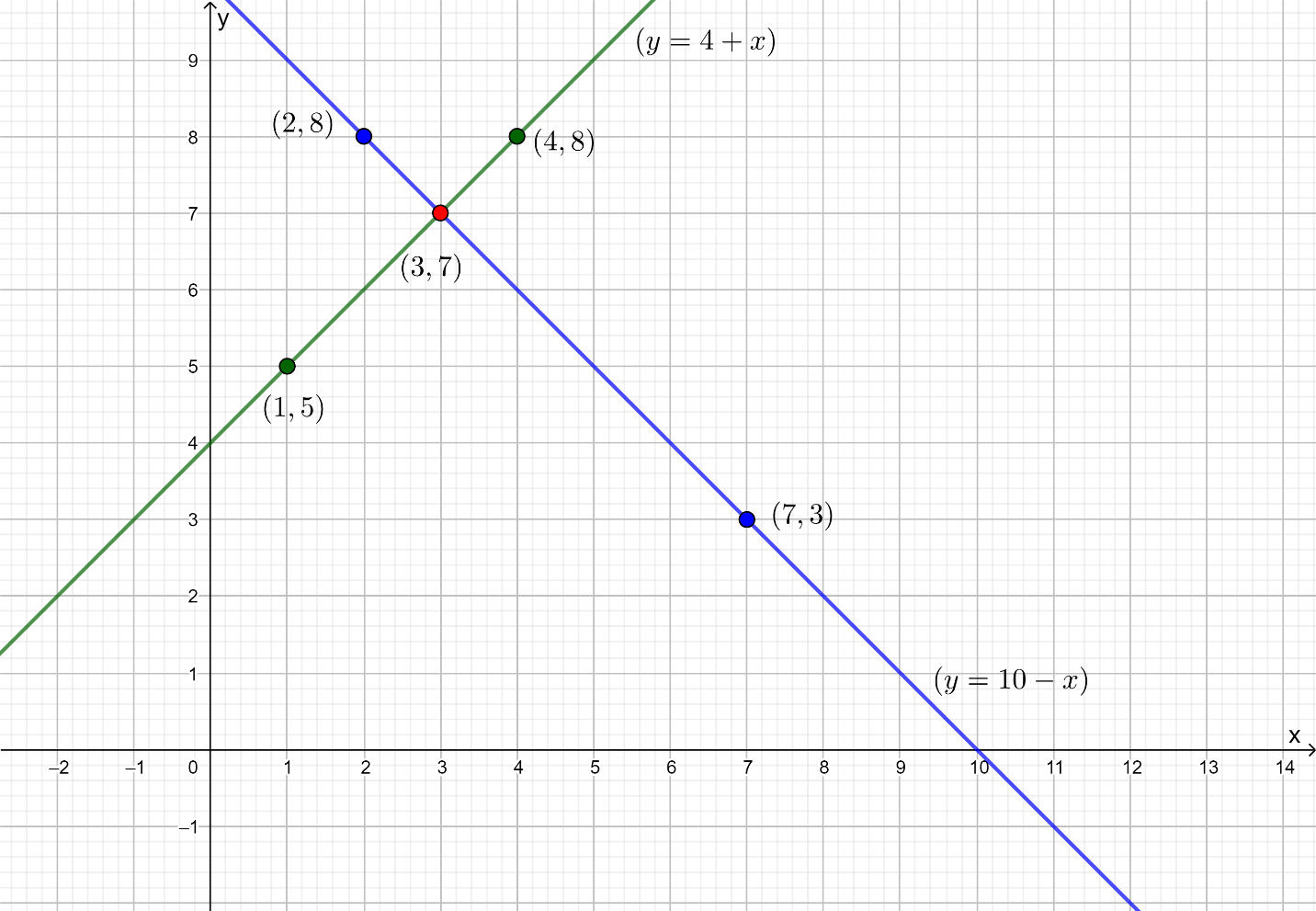Answer:

From graph solution $$\left( {x,y} \right) = \left( {3,7} \right)$$

Number of boys $$= 3$$

Number of girls$$= 7$$

(ii)

What is Known?

(i) $$5$$ pencils and $$7$$ pens cost ₹ $$50$$ $$\left( {x,y} \right) = \left( {3,7} \right)$$

(ii) $$7$$ pencils and $$5$$ pens cost ₹ $$46$$

What is Unknown?

Finding Solutions graphically for the given situation.

Reasoning:

Assuming the cost of $$1$$ pencil as ₹ $$x$$ and the cost of $$1$$ pen as ₹ $$y,$$ two linear equations are to be formed for the above situation.

Steps:

Let us assume cost of $$1$$ pencil be $$x$$ and cost of $$1$$ pen be $$y.$$

The cost of $$5$$ pencils and $$7$$ pens is ₹ $$50.$$

Mathematically,

$5x + 7y = 50$

And, the cost of $$7$$ pencils and 5 pens is ₹ $$50.$$

Mathematically,

$7x + 5y = 46$

Algebraic representation where $$x$$ and $$y$$ are the cost of $$1$$ pencil and $$1$$ pen respectively.

\begin{align}5x + 7y &= 50 \,\dots (1) \\7x + 5y &= 46 \,\dots(2) \end{align}

Therefore, the algebraic representation for equation $$1$$ is:

\begin{align}5x+ 7y &= 50\\7y &= 50-5x\\y &= \frac{{50 - 5x}}{7}\end{align}

And, the algebraic representation for equation $$2$$ is:

\begin{align}7x + 5y &= 46\\5y &= 46-7x\\y &= \frac{{46 - 7x}}{5}\end{align}

Let us represent these equations graphically. For this, we need at least two solutions for each equation. We give these solutions in table shown below.

 $$x$$ $$3$$ $$- 4$$ $$y = \frac{{50 - 5x}}{7}$$ $$5$$ $$10$$

 $$x$$ $$3$$ $$8$$ $$y = \frac{{46 - 7x}}{5}$$ $$5$$ $$- 2$$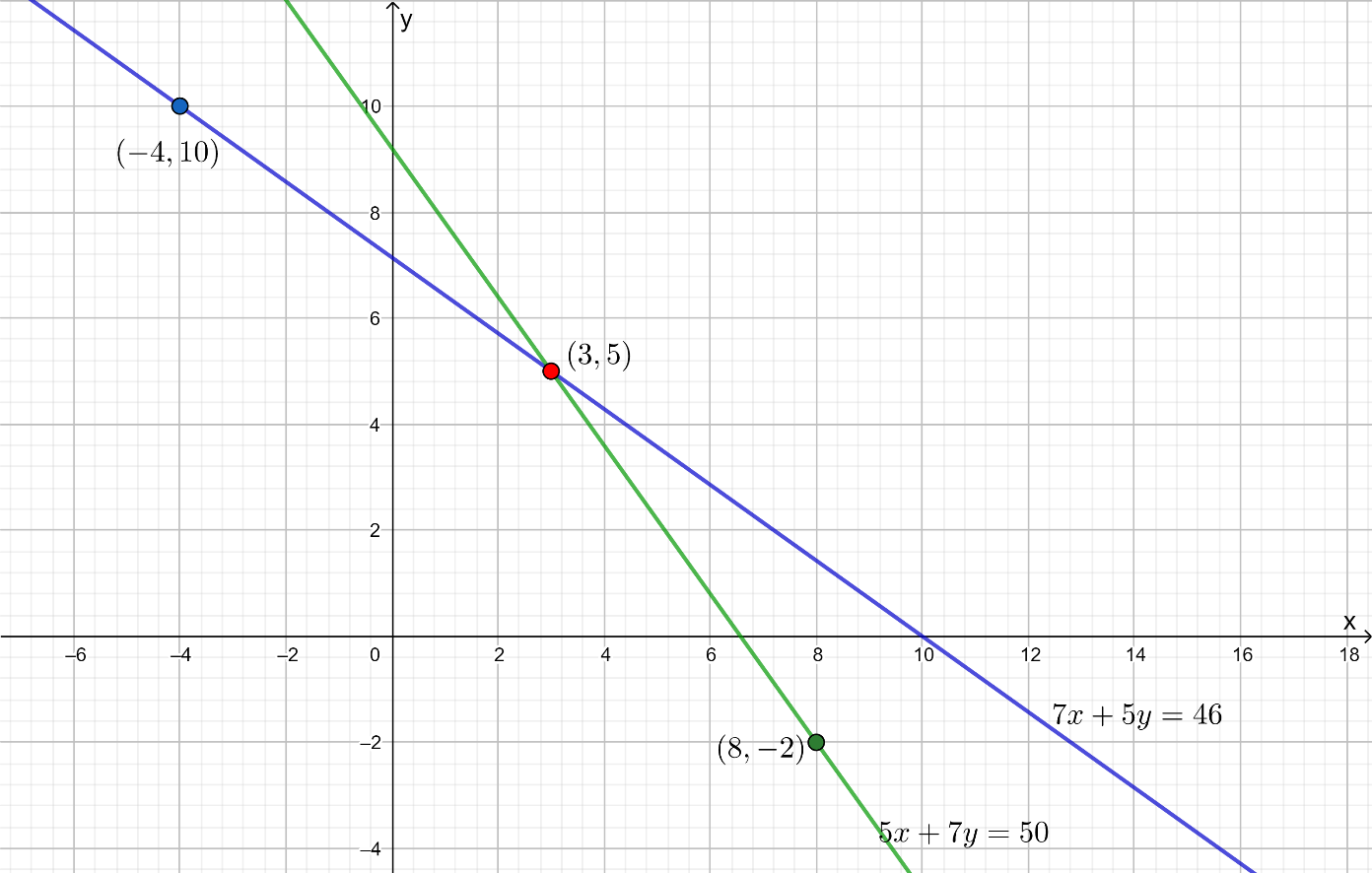From graph Solution $$\left( {x,y} \right) = \left( {3,5} \right)$$

Cost of one pencil $$=$$$$3$$

Cost of one pen $$=$$$$5$$

## Chapter 3 Ex.3.2 Question 2

On comparing the ratios \begin{align}\frac{{{a_1}}}{{{a_2}}} = \frac{{{b_1}}}{{{b_2}}} = \frac{{{c_1}}}{{{c_2}}}, \end{align} find out whether the lines representing the following pairs of linear equations intersect at a point, are parallel or coincident:

(i)

\begin{align}\quad 5x-4y + 8 &= 0\\7x + 6y-9 &= 0\end{align}

(ii)

\begin{align}\quad 9x + 3y + 12& = 0\\18x + 6y + 24 &= 0\end{align}

(iii)

\begin{align}\quad 6x-3y + 10 &= 0\\2x-y + 9 &= 0\end{align}

### Solution

(i) What is Known?

\begin{align}5x-4y + 8 &= 0\\7x + 6y-9 &= 0\end{align}

What is Unknown?

Whether the lines are

(i) Intersecting

(ii) Parallel

(iii) Coincident

Reasoning:

For any pair of linear equation

$$a_1 x + b_1 y + c_1 = 0\\ a_2 x + b_2 y + c_2 = 0$$

a) \begin{align}\frac{{{a_1}}}{{{a_2}}} \ne \frac{{{b_1}}}{{{b_2}}}\end{align} (Intersecting Lines)

b)  \begin{align}\frac{{{a_1}}}{{{a_2}}} = \frac{{{b_1}}}{{{b_2}}} = \frac{{{c_1}}}{{{c_2}}}\end{align} (Coincident Lines)

c)  \begin{align}\frac{{{a_1}}}{{{a_2}}} = \frac{{{b_1}}}{{{b_2}}} \ne \frac{{{c_1}}}{{{c_2}}}\end{align} (Parallel Lines)

Steps:

\begin{align}{a_1} &= 5 \;\,\qquad{b_1} = - 4 \qquad {c_1} = 8\\{a_2} &= 7 \qquad \;\,{b_2} = 6 \;\;\;\qquad {c_2} = - 9\end{align}

\begin{align}\frac{{{a_1}}}{{{a_2}}} &= \frac{5}{7} \qquad \;\;\; \dots(1)\\ \frac{{{b_1}}}{{{b_2}}} = \frac{{ - 4}}{6} &= \frac{{ - 2}}{3} \qquad \dots (2)\end{align}

From (i) and (ii)

$\frac{{{a_1}}}{{{a_2}}} \ne \frac{{{b_1}}}{{{b_2}}}$

Therefore, they are intersecting lines at a point

(ii) What is Known?

\begin{align}9x + 3y + 12& = 0\\18x + 6y + 24 &= 0\end{align}

What is Unknown?

Whether the lines are

(i) Intersecting

(ii) Parallel

(iii) Coincident

Reasoning:

For any pair of linear equation

\begin{align}{a_1}x + {b_1}y + {c_1} &= 0\\{a_2}x + {b_2}y + {c_2} &= 0\end{align}

a) \begin{align}\frac{{{a_1}}}{{{a_2}}} \ne \frac{{{b_1}}}{{{b_2}}}\end{align}(Intersecting Lines)

b) \begin{align}\frac{{{a_1}}}{{{a_2}}} = \frac{{{b_1}}}{{{b_2}}} = \frac{{{c_1}}}{{{c_2}}}\end{align}(Coincident Lines)

c) \begin{align}\frac{{{a_1}}}{{{a_2}}} = \frac{{{b_1}}}{{{b_2}}} \ne \frac{{{c_1}}}{{{c_2}}}\end{align}(Parallel Lines)

Steps:

\begin{align}{a_1} &= 9, \quad {b_1} = 3 \quad {c_1} = 12\\{a_2}& = 18\quad {b_2} = 6\quad {c_2} = 24\end{align}

\begin{align}\frac{{{a_1}}}{{{a_2}}} &= \frac{9}{{18}} = \frac{1}{2} \quad \dots(1)\\ \frac{{{b_1}}}{{{b_2}}} &= \frac{3}{6} = \frac{1}{2} \quad \;\;\dots(2)\\ \frac{{{c_1}}}{{{c_2}}} &= \frac{{12}}{{24}} = \frac{1}{2} \quad \dots(3) \end{align}

From (1), (2) and (3)

$\frac{{{a_1}}}{{{a_2}}} = \frac{{{b_1}}}{{{b_2}}} = \frac{{{c_1}}}{{{c_2}}} = \frac{1}{2}$

Therefore, they are coincident lines

(iii) what is Known?

\begin{align}6x-3y + 10 &= 0\\2x-y + 9 &= 0\end{align}

What is Unknown?

Whether the lines

(i) Intersecting

(ii) Parallel

(iii) Coincident

Reasoning:

For any pair of linear equation

\begin{align}{a_1}x + {b_1}y + {c_1} = 0\\{a_2}x + {b_2}y + {c_2} = 0\end{align}

a) \begin{align}\frac{{{a_1}}}{{{a_2}}} \ne \frac{{{b_1}}}{{{b_2}}}\end{align} (Intersecting Lines)

b) \begin{align}\frac{{{a_1}}}{{{a_2}}} = \frac{{{b_1}}}{{{b_2}}} = \frac{{{c_1}}}{{{c_2}}}\end{align} (Coincident Lines)

c) \begin{align}\frac{{{a_1}}}{{{a_2}}} = \frac{{{b_1}}}{{{b_2}}} \ne \frac{{{c_1}}}{{{c_2}}}\end{align} (Parallel Lines)

Steps:

\begin{align}{a_1} &= 6,\quad {b_1} = - 3\quad{c_1} = 10\\{a_2} &= 2 \quad \; {b_2} = - 1 \quad \,{c_2} = 9\end{align}

\begin{align}\frac{{{a_1}}}{{{a_2}}}& = \frac{6}{2} = 3 \qquad \quad \dots(1)\\\frac{{{b_1}}}{{{b_2}}} &= \frac{{ - 3}}{{ - 1}} = 3 \qquad \dots(2)\\\frac{{{c_1}}}{{{c_2}}} &= \frac{{10}}{9} \qquad \qquad \;\dots(3)\end{align}

From $$(1), (2)$$ and $$(3)$$

$\frac{{{a_1}}}{{{a_2}}} = \frac{{{b_1}}}{{{b_2}}} \ne \frac{{{c_1}}}{{{c_2}}}$

Therefore, they are parallel lines.

## Chapter 3 Ex.3.2 Question 3

On comparing the ratios \begin{align}\frac{{{a_1}}}{{{a_2}}},\frac{{{b_1}}}{{{b_2}}},\frac{{{c_1}}}{{{c_2}}}\end{align}, find out whether the following pair of linear equations are consistent, or inconsistent.

(i)

$$3x + 2y = 5;\;\;2x-3y = 7$$

(ii)

$$2x-3y = 8;\;\;4x-6y = 9$$

(iii)

\begin{align} \frac{3}{2}x + \fracabc{3}y = 7;\;\;9x-10y = 14 \end{align}

(iv)

$$5x-3y = 11;\;\;-10x + 6y = -22$$

(v)

\begin{align} \frac{4}{3}x + 2y = 8;\;\;2x + 3y = 12 \end{align}

### Solution

What is Unknown?

To find out whether the linear equations are consistent or inconsistent.

Reasoning:

For any pair of linear equation

\begin{align}{a_1}x + {b_1}y + {c_1} &= 0\\{a_2}x + {b_2}y + {c_2} &= 0\end{align}

Consistent means pair of linear equations have one solution or infinitely many solutions.

\begin{align}&\frac{{{a_1}}}{{{a_2}}} \ne \frac{{{b_1}}}{{{b_2}}}\\& \begin{bmatrix} \text{Intersecting lines/one} \\ \text{Solution} \end{bmatrix} \\&\,\frac{{{a_1}}}{{{a_2}}} = \frac{{{b_1}}}{{{b_2}}} = \frac{{{c_1}}}{{{c_2}}}\\&\left[ \begin{array} \ {\text{Coincident Lines/ Infinitely}}\\{\text{ many Solutions}} \end{array}\right]\end{align}

Inconsistent means, the lines may be parallel and do not have any Solution)

\begin{align}\frac{{{a_1}}}{{{a_2}}} = \frac{{{b_1}}}{{{b_2}}} \ne \,\,\frac{{{c_1}}}{{{c_2}}}\end{align} (Parallel lines/No Solution)

(i) What is Known?

\begin{align}3x + 2y - 5 &= 0{\rm{ }}\\2x-3y - 7 &= 0\end{align}

Steps:

\begin{align}\frac{{{a_1}}}{{{a_2}}} &= \frac{3}{2}\\\frac{{{b_1}}}{{{b_2}}} &= \frac{2}{{ - 3}}\\ \frac{{{c_1}}}{{{c_2}}} &= \frac{{ - 5}}{{ - 7}} \\&= \frac{5}{7}\end{align}

From above

$\frac{{{a_1}}}{{{a_2}}} \ne \frac{{{b_1}}}{{{b_2}}}$

Therefore, lines are intersecting and have one solution,

Hence, the pair of equations are consistent.

(ii) What is Known:

\begin{align}2x-3y - 8 &= 0\\4x-6y - 9 &= 0\end{align}

Steps:

\begin{align}\frac{{{a_1}}}{{{a_2}}} &= \frac{2}{4} = \frac{1}{2}\\\frac{{{b_1}}}{{{b_2}}} &= \frac{{ - 3}}{{ - 6}} = \frac{1}{2}\\\frac{{{c_1}}}{{{c_2}}} &= \frac{{ - 8}}{{ - 9}} = \frac{8}{9}\end{align}

From above

$\frac{{{a_1}}}{{{a_2}}} = \frac{{{b_1}}}{{{b_2}}} \ne \,\,\frac{{{c_1}}}{{{c_2}}}\,$

Therefore, lines are parallel and have no solution,

Hence, the pair of equations are inconsistent.

(iii) What is Known?

\begin{align}\frac{3}{2}x + \frac{5}{3}y &= 7{\rm{ }}\\9x - 10y &= 14\end{align}

Steps:

\begin{align}\frac{{{a_1}}}{{{a_2}}} &= \frac{{\frac{3}{2}}}{9} \\&= \frac{3}{2} \times \frac{1}{9}\\& = \frac{1}{6}\\ \frac{{{b_1}}}{{{b_2}}} &= \frac{{\frac{5}{3}}}{{ - 10}} \\&= \frac{5}{3} \times \frac{1}{{ - 10}}\\& = \frac{1}{{ - 6}}\\ \frac{{{c_1}}}{{{c_2}}} &= \frac{7}{{14}} \\&= \frac{1}{2}\end{align}

From above

$\frac{{{a_1}}}{{{a_2}}} \ne \frac{{{b_1}}}{{{b_2}}}\,$

Therefore, lines are intersecting and have one solution.

Hence, they are consistent.

(iv) What is Known?

\begin{align}5x-3y - 11 &= 0{\rm{ }}\\-10x + 6y + 22 &= 0\end{align}

Steps:

\begin{align}\frac{{{a_1}}}{{{a_2}}} &= \frac{5}{{ - 10}} \\&= \frac{{ - 1}}{2}\\ \frac{{{b_1}}}{{{b_2}}} &= \frac{{ - 3}}{6} \\&= \frac{{ - 1}}{2}\\\frac{{{c_1}}}{{{c_2}}}& = \frac{{ - 11}}{{22}} \\&= \frac{{ - 1}}{2}\end{align}

From above

$\frac{{{a_1}}}{{{a_2}}} = \frac{{{b_1}}}{{{b_2}}} = \,\,\frac{{{c_1}}}{{{c_2}}}\,$

Therefore, lines are coincident and have infinitely many solutions.

Hence, they are consistent.

(v) What is Known?

\begin{align}\frac{4}{3}x + 2y &= 8\\2x + 3y &= 12\end{align}

Steps:

\begin{align}\frac{{{a_1}}}{{{a_2}}}& = \frac{{\frac{4}{3}}}{2} = \frac{4}{3} \times \frac{1}{2} \\&= \frac{2}{3}\\ \frac{{{b_1}}}{{{b_2}}} &= \frac{2}{3}\\\frac{{{c_1}}}{{{c_2}}} &= \frac{{ - 8}}{{ - 12}}\\& = \frac{2}{3}\end{align}

From above

$\frac{{{a_1}}}{{{a_2}}} = \frac{{{b_1}}}{{{b_2}}} = \frac{{{c_1}}}{{{c_2}}}\,$

Therefore, lines are coincident and have infinitely many solutions.

Hence, they are consistent.

## Chapter 3 Ex.3.2 Question 4

Which of the following pairs of linear equations are consistent / inconsistent? If consistent, obtain the Solution graphically:

(i)

$$x + y = 5, \;2x + 2y = 10$$

(ii)

$$x-y = 8, \;3x-3y = 16$$

(iii)

$$2x + y-6 = 0, \;4x-2y-4 = 0$$

(iv)

$$2x-2y-2 = 0, \;4x-4y-5 = 0$$

### Solution

What is Unknown?

Whether the linear equations are consistent or inconsistent and graphical solution, if consistent.

Reasoning:

Consistent means pair of linear equations have one solution or infinitely many solutions.

\begin{align}{a_1}x + {b_1}y + {c_1} &= 0\\{a_2}x + {b_2}y + {c_2} &= 0\end{align}

\begin{align}&\frac{{{a_1}}}{{{a_2}}} \ne \frac{{{b_1}}}{{{b_2}}}\\& \begin{bmatrix} {\text{Intersecting lines/one}}\\ {\text{Solution}} \end{bmatrix} \\&\,\frac{{{a_1}}}{{{a_2}}} = \frac{{{b_1}}}{{{b_2}}} = \frac{{{c_1}}}{{{c_2}}}\\&\left[ \begin{array} \ {\text{Coincident Lines/Infinitely}}\\{\text{ many Solutions}} \end{array}\right]\end{align}

Inconsistent means, the lines may be parallel and do not have any Solution)

$$\frac{{{a_1}}}{{{a_2}}} = \frac{{{b_1}}}{{{b_2}}} \ne \,\,\frac{{{c_1}}}{{{c_2}}}$$ (Parallel lines/ No Solution)

(i) What is Known?

\begin{align}x + y-5 &= 0\\2x + 2y-10 &= 0\end{align}

Steps:

\begin{align}\frac{{{a_1}}}{{{a_2}}} &= \frac{1}{2}\\\frac{{{b_1}}}{{{b_2}}} &= \frac{1}{2}\\\frac{{{c_1}}}{{{c_2}}} &= \frac{{ - 5}}{{ - 10}} \\&= \frac{1}{2}\end{align}

From above

$\frac{{{a_1}}}{{{a_2}}} = \frac{{{b_1}}}{{{b_2}}} = \,\,\frac{{{c_1}}}{{{c_2}}}$

Therefore, lines are coincident and have infinitely many solutions.

Hence, they are consistent.

\begin{align}x + y-5 &= 0\\y &= - x + 5\\y& = 5-x\end{align}

 $$x$$ $$1$$ $$2$$ $$y = 5-x$$ $$4$$ $$3$$

\begin{align}2x + 2y-10 &= 0\\2y &= 10-2x\\y &= 5-x\end{align}

 $$x$$ $$3$$ $$4$$ $$y = 5-x$$ $$2$$ $$1$$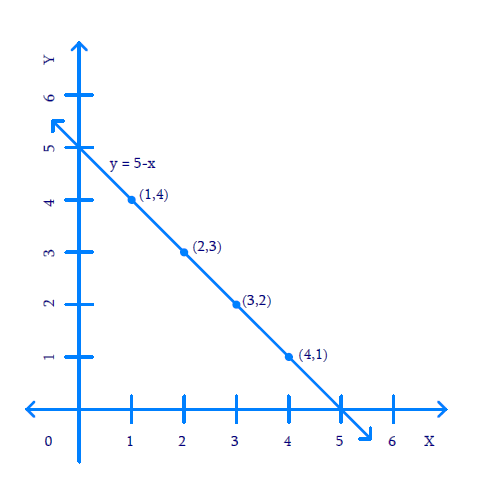All the points on coincident line are solutions for the given pair of equations.

(ii) What is Known?

\begin{align}x-y-8 &= 0\\3x-3y-16 &= 0\end{align}

Steps:

\begin{align}\frac{{{a_1}}}{{{a_2}}} &= \frac{1}{3}\\\frac{{{b_1}}}{{{b_2}}} &= \frac{{ - 1}}{{ - 3}}\\&= \frac{1}{3}\\\frac{{{c_1}}}{{{c_2}}} &= \frac{{ - 8}}{{ - 16}} \\&= \frac{1}{2}\end{align}

From above

$\frac{{{a_1}}}{{{a_2}}} = \frac{{{b_1}}}{{{b_2}}} \ne \,\,\frac{{{c_1}}}{{{c_2}}}\,$

Therefore, lines are parallel and have no solution,

Hence, the pair of equations are inconsistent.

\begin{align}x - y - 8 &= 0\\y &= x - 8\end{align}

 $$x$$ $$8$$ $$6$$ $$y{\rm{ }} = {\rm{ }}x{\rm{ }}-{\rm{ }}8$$ $$0$$ $$- 2$$

\begin{align}3x - 3y - 16 &= 0\\3y &= 3x - 16\\y &= \frac{{3x - 16}}{3}\end{align}

 $$x$$ $$2$$ $$4$$ $$y = \frac{{3x - 16}}{3}$$ $$- 3.3$$ $$- 1.3$$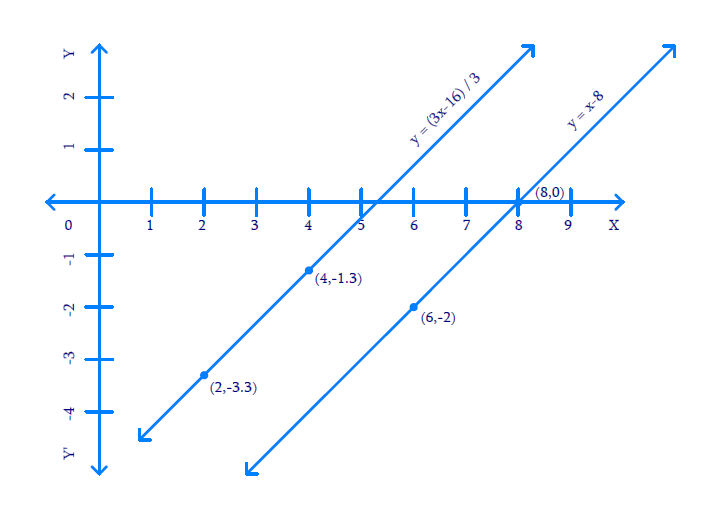(iii) What is Known?

\begin{align}2x + y-6 &= 0\\4x-2y-4 &= 0\end{align}

Steps:

\begin{align}\frac{{{a_1}}}{{{a_2}}} &= \frac{2}{4}\\& = \frac{1}{2}\\\frac{{{b_1}}}{{{b_2}}} &= \frac{1}{{ - 2}} \\&= - \frac{1}{2}\\\frac{{{c_1}}}{{{c_2}}} &= \frac{{ - 6}}{{ - 4}} \\&= \frac{3}{2}\end{align}

From above:

$\frac{{{a_1}}}{{{a_2}}} \ne \frac{{{b_1}}}{{{b_2}}}\,$

Therefore, lines are intersecting and have one solution.

Hence, they are consistent.

\begin{align}2x + y-6 &= 0\\y &= 6-2x\end{align}

 $$x$$ $$0$$ $$2$$ $$y = 6-2x$$ $$6$$ $$2$$

\begin{align}4x - 2y - 4 &= 0\\2y &= 4x - 4\\y& = 2x - 2\end{align}

 $$x$$ $$2$$ $$3$$ $$y = 2x - 2$$ $$2$$ $$4$$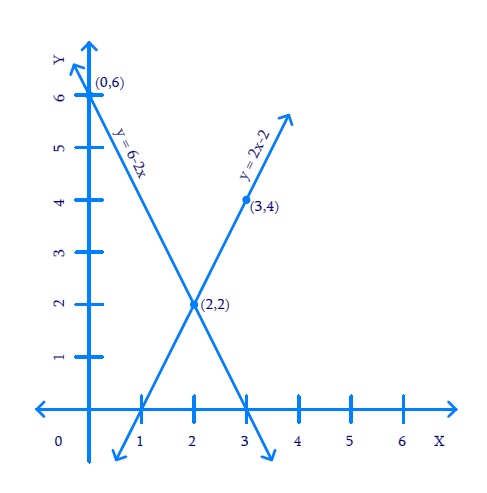$$x=2$$ and $$y=2$$ are solutions for the given pair of equations.

(iv) What is Known?

\begin{align}2x-2y-2 &= 0\\4x-4y-5& = 0 \\\end{align}

Steps:

\begin{align}\frac{{{a_1}}}{{{a_2}}} &= \frac{2}{4} \\&= \frac{1}{2}\\\frac{{{b_1}}}{{{b_2}}} &= \frac{{ - 2}}{{ - 4}}\\&= \frac{1}{2}\\\frac{{{c_1}}}{{{c_2}}} &= \frac{{ - 2}}{{ - 5}}\\& = \frac{2}{5}\end{align}

From above:

$\frac{{{a_1}}}{{{a_2}}} = \frac{{{b_1}}}{{{b_2}}} \ne \,\,\frac{{{c_1}}}{{{c_2}}}\,$

Therefore, lines are parallel and have no solution,

Hence, the pair of equations are inconsistent.

\begin{align}2x - 2y - 2 &= 0\\2y &= 2x - 2\\y &= x - 1\end{align}

 $$x$$ $$1$$ $$3$$ $$y = x-1$$ $$0$$ $$2$$

\begin{align}4x - 4y - 5 &= 0\\4y &= 4x - 5\\y& = \frac{{4x - 5}}{4}\end{align}

 $$x$$ $$4$$ $$3$$ $$y = \frac{{4x - 5}}{4}$$ $$2.8$$ $$1.8$$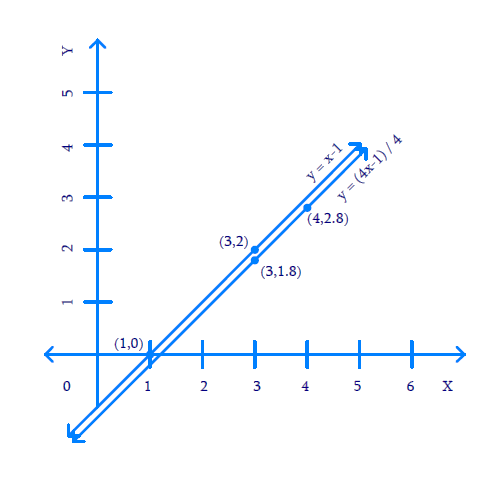## Chapter 3 Ex.3.2 Question 5

Half the perimeter of a rectangular garden, whose length is $$4 \,\rm{m}$$ more than its width, is $$36\,\rm{ m.}$$ Find the dimensions of the garden.

### Solution

What is Known?

(i) Half the perimeter of rectangular garden $$= 36{\rm{\,m }}$$

(ii) Length is $$4{\rm{ \,m}}$$ more than width

What is Unknown?

Dimensions of the garden

Reasoning:

Assuming length of the garden as $$x$$ and width of the garden as $$y,$$ two linear equations can be formed for the known data.

Perimeter of rectangle $$=$$ 2(Length $$+$$ Breadth)

Steps:

Let the length of the garden be $$x$$ and breadth be $$y$$

Then,

\begin{align}x& = y + 4\\ x - y &= 4\\y &= x - 4\end{align}

 $$x$$ $$8$$ $$16$$ $$y = x - 4$$ $$4$$ $$12$$

Half perimeter of the rectangle be $$x + y = 36$$

$$y = 36 - x$$

 $$x$$ $$16$$ $$26$$ $$y = x - 4$$ $$20$$ $$10$$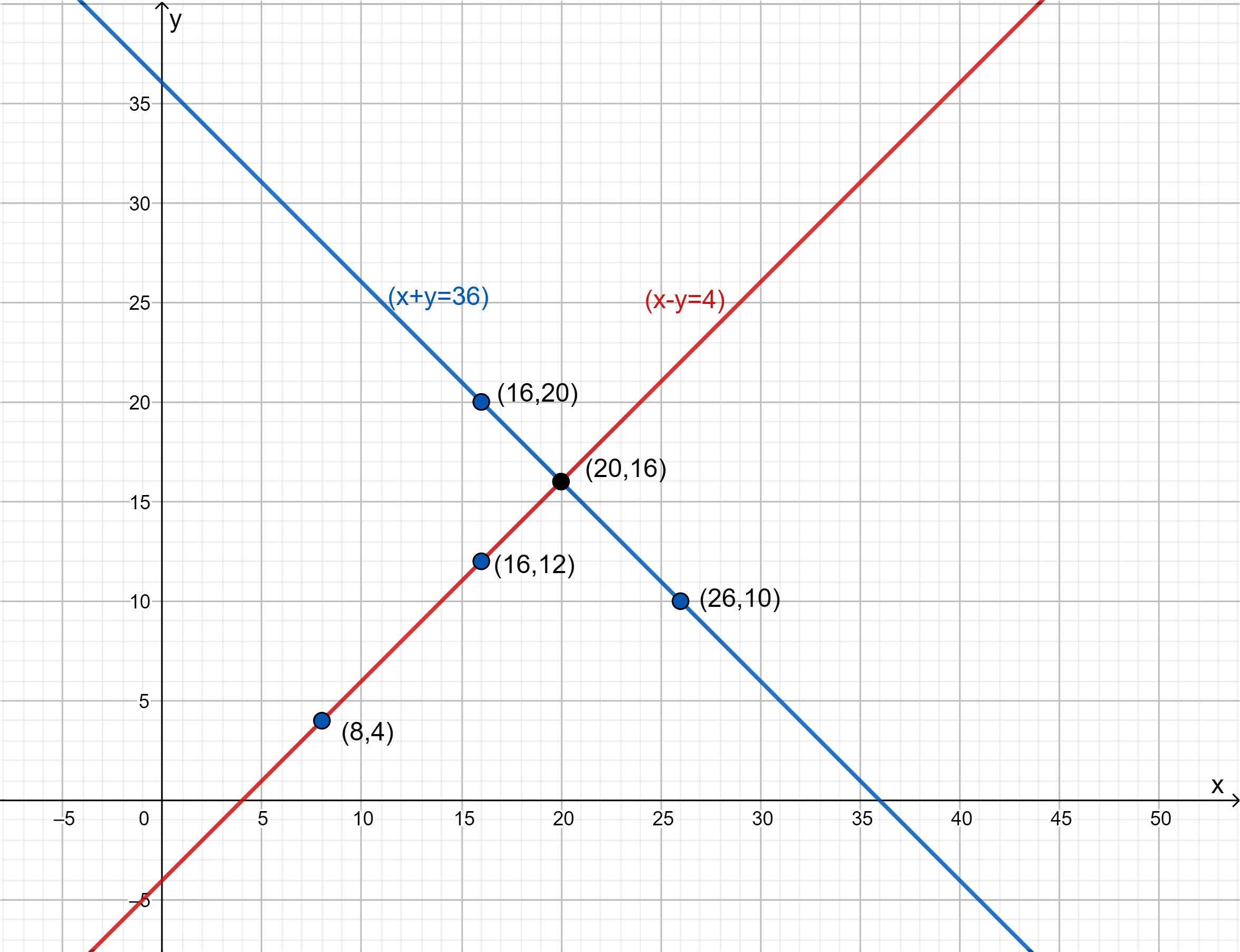The answer is

Length $$x = 20{\rm{\; m}}$$

Breadth $$y = 16{\rm{\; m}}$$

## Chapter 3 Ex.3.2 Question 6

Given the linear equation $$2x + 3y-8 = 0,$$ write another linear equation in two variables such that the geometrical representation of the pair so formed is:

(i) intersecting lines

(ii) parallel lines

(iii) coincident lines

### Solution

What is Known?

One linear equation $$2x + 3y-8 = 0$$

What is Unknown?

Another linear equation such that given is satisfied.

Reasoning:

Same as Exercise 3.2 (2)

(i) Intersecting lines

Condition: \begin{align} \frac{{{a_1}}}{{{a_2}}} \ne \,\frac{{{b_1}}}{{{b_2}}}\, \end{align}

\begin{align}2x + 3y-8 &= 0\\{a_1}& = 2\\{b_1} &= 3\end{align}

So, considering $${a_2} = 3$$ and $${b_2} = 2$$ will satisfy the condition for intersecting lines $${c_2}$$ can be any value.

\begin{align}\frac{{{a_1}}}{{{a_2}}} &= \frac{2}{3} \quad \frac{{{b_1}}}{{{b_2}}} = \frac{3}{2}\\\frac{2}{3} &\ne \frac{3}{2}\,\end{align}

$$\therefore$$ Another linear equation is

$$3x + 2y-6 = 0$$

(ii) Parallel Lines

Condition: \begin{align} \frac{{{a_1}}}{{{a_2}}} = \,\frac{{{b_1}}}{{{b_2}}} \ne \frac{{{c_1}}}{{{c_2}}} \end{align}

\begin{align}2x + 3y-8 &= 0\\{a_1} &= 2\\{b_1} &= 3\\{c_1} &= - 8\end{align}

So, considering $${a_2} = 4,\;\;{b_2} = 6,\;\;{c_2} = 9$$ will satisfy the condition for parallel lines.

\begin{align}\frac{{{a_1}}}{{{a_2}}}&= \frac{2}{4} = \frac{1}{2}\\\frac{{{b_1}}}{{{b_2}}} &= \frac{3}{6} = \frac{1}{2}\\\frac{{{c_1}}}{{{c_2}}}& = \frac{{ - 8}}{9}\end{align}

From above:

$\frac{{{a_1}}}{{{a_2}}} = \,\frac{{{b_1}}}{{{b_2}}} \ne \frac{{{c_1}}}{{{c_2}}}$

Therefore, another linear equation is

$$= 4x + 6y + 9 = 0$$

(iii) Coincident lines:

Condition: \begin{align} \frac{{{a_1}}}{{{a_2}}} = \,\frac{{{b_1}}}{{{b_2}}} = \frac{{{c_1}}}{{{c_2}}} \end{align}

\begin{align}2x + 3y-8 &= 0\\{a_1} &= 2\\{b_1} &= 3\\{c_1}& = - 8\end{align}

So, considering $${a_2} = 4,\;\;{b_2} = 6,\;\;{c_2} = - 16$$ will satisfy the condition for parallel lines.

\begin{align}\frac{{{a_1}}}{{{a_2}}} &= \frac{2}{4} = \frac{1}{2}\\\frac{{{b_1}}}{{{b_2}}} &= \frac{3}{6} = \frac{1}{2}\\\frac{{{c_1}}}{{{c_2}}} &= \frac{{ - 8}}{{ - 16}} = \frac{1}{2}\end{align}

From above:

$\frac{{{a_1}}}{{{a_2}}} = \,\frac{{{b_1}}}{{{b_2}}} = \frac{{{c_1}}}{{{c_2}}}$

Therefore, linear equation is

$$4x + 6y-16 = 0$$

## Chapter 3 Ex.3.2 Question 7

Draw the graphs of the equations $$x-y + 1 = 0$$ and $$3x + 2y-12 = 0.$$ Determine the coordinates of the vertices of the triangle formed by these lines and the $$x-$$axis and shade the triangular region.

### Solution

What is Known?

Linear equation

\begin{align}x - y + 1 &= 0\\3x + 2y-12 &= 0\end{align}

What is Unknown?

Coordinates of the vertices of the triangle formed by intersecting lines and the $$x$$-axis

Reasoning:

From graph of two linear equations and $$x$$-axis, triangle can be shaded, and vertices can be located.

Steps:

\begin{align}x - y + 1 &= 0\\y &= x + 1\end{align}

 $$x$$ $$0$$ $$1$$ $$y = x + 1$$ $$1$$ $$2$$

\begin{align}3x + 2y-12 &= 0\\2y &= 12 - 3x\\y &= \frac{{12 - 3x}}{2}\end{align}

 $$x$$ $$0$$ $$2$$ $$y = \frac{{12 - 3x}}{2}$$ $$6$$ $$3$$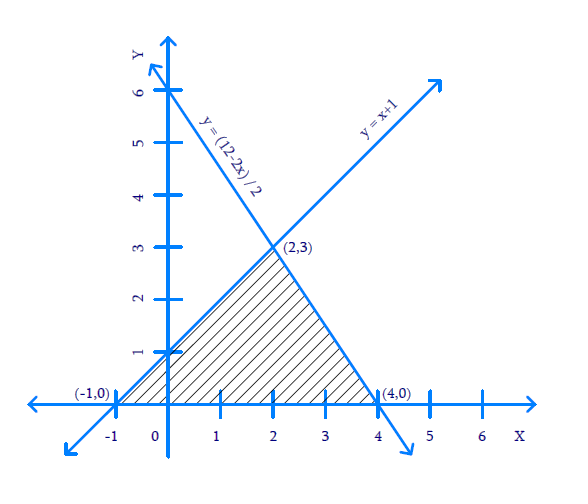From graph, Vertices are $$(-1, \,0),\; (4,\, 0),$$ and $$(2, \,3)$$

Download SOLVED Practice Questions of NCERT Solutions For Class 10 Maths Chapter 3 Exercise 3.2 for FREE
Ncert Class 10 Exercise 3.2
Ncert Solutions For Class 10 Maths Chapter 3 Exercise 3.2
Instant doubt clearing with Cuemath Advanced Math Program